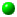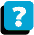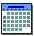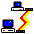Module 1.4 Notes "Estimating with Confidence"

 Index to Module One Notes1.1: Why Statistics for Mgt1.2: Describing Data: Pictures1.3: Describing Data: Number Summaries1.4: Estimating with Confidence1.5: Testing Hypothesis

In Module 1.2 and 1.3, we started with a set of data (it could be a sample or a population) and described the shape, center, spread, noise and signals of that set of data with pictures and number summaries. Describing data we have is called descriptive statistics.

Introduction to Confidence Interval Estimation

Inferential statistics starts with a research question or hypothesis about a population parameter, such as a mean or a proportion. The research question may be as simple as "What is the true population mean cycle time for this company?" Next, we gather data in a (hopefully) unbiased sample without measurement error. Levine, Berenson and Stephan (Chapter 1, 1999) present four primary ways of gathering data:

2. Design an experiment to obtain the necessary data.

3. Conduct a survey.

4. Conduct an observational survey.

For estimating cycle time, we would probably conduct an observational survey. We may try to obtain industry data for benchmark purposes, but to understand the true mean cycle time facing this particular company, observations for that company must be made.

The sample we studied in Modules 1.2 and 1.3 consists of 30 observations of monthly cycle times. We want to use this sample to estimate the true population mean cycle time. Note that this sample consists of 30 consecutive months. If there was a seasonal factor we would need to stratify the data into groups that have like seasonal patterns. The interested reader is referred to the reference for more discussion on data collection and sampling.

The final step is to use sample statistics (such as the sample mean) and an appropriate measure of reliability to make an inference about the population parameter. The two methods of inferential statistics are confidence interval estimation and tests of hypothesis. Tests of hypothesis will be covered in Module 1.5.

The Concept
To estimate the value of the population mean, we start with a point estimate. The most efficient and unbiased point estimate of the population mean is the sample mean. So:

Equation (Eq.) 1.4.1: Population Mean = Sample Mean

But a sample mean represents the center of just one sample. If we took another sample, we would get another sample mean, probably close to the first one, but nonetheless different since the chance of getting exactly the same measure of center from different samples is very, very rare. If we take another sample, we get another sample mean. In fact, if we take enough samples to get many sample means, we can construct a probability distribution for the sample mean with its own mean and standard deviation. A little more on this latter. For now, the point is that when estimating the population mean with a sample mean, we have to recognize that there is going to be sampling error since we are working with a sample and not the population. So we get a much richer and accurate estimate of the population mean by incorporating sampling error, as our measure of reliability, into the equation:

Eq. 1.4.2: Population Mean = Sample Mean + Sampling Error

The interval, sample mean + sampling error, is called a confidence interval for the population mean. The interval is also know as the interval estimate of the population mean. Sampling error is a function of how much confidence we want in our estimate (typically a 95% or 99% confidence level), the standard deviation of the set of data, and the sample size. Let's look at our first confidence interval, and use it to explain more about sampling error.

Large Sample Confidence Interval for the Population Mean with Known Population Standard Deviation

This confidence interval is:

Eq. 1.4.3: (1 - alpha)% Confidence Interval for True Population Mean
= Sample Mean + Z *[Pop. Std. Dev./Sq. Rt. (n)], where
Pop. Std. Dev. = Population Standard Deviation, and
Sq. Rt. (n) = Square Root of sample size n

The quantity (1 - alpha)% represents how much confidence we want in estimating the true population mean, again, typically 95% or 99%. If we wanted to be 95% confident in estimating the population mean, which is similar to saying that we want the probability of capturing the true population mean to be 95%, then Z takes on the value of 1.96 (or 2 by rounding). Alpha, in the expression (1 - alpha), takes on the value 0.05, and represents the probability of NOT capturing the population mean in repeated sampling.

Let's incorporate the above discussion into a new equation:

Eq. 1.4.4: 95% Confidence Interval for True Population Mean
= Sample Mean + 1.96 * [(Pop. Std. Dev./Sq. Rt.(n)]

The next expression, [Pop. Std. Dev./Sq. Rt.(n), is called the standard error of the mean. It is similar to the standard deviation in that it measures average deviation, but is different in what deviation is being measured.

Pause and Reflect:
The standard deviation is the average manner in which observations vary around the sample mean.
The interpretation is given with respect to the mean: 95% (or most) of the observations in symmetric bell-shaped distribution fall between the mean + 2 * standard deviation.

On the other hand, the standard error of the mean is the average manner in which sample means vary around the population mean. The formula for the standard error of the mean is derived from the theoretical sampling distribution of the mean. I won't be going into the theory, but it can be shown that the sampling distribution of the mean is a normal distribution with the mean equal to the population mean, and the standard deviation equal to the population standard deviation divided by the square root of the sample size. This is true even if the population is not normally distributed, as long as the sample size is large enough (at least 30); or if the population is approximately normal, as long as the sample size is at least 15. The theorem is called the central limit theorem, and the interested reader is to pp. 373-387 in the reference text for more details.

Back to our derivation of the confidence interval. Suppose we know that the sample mean is 21, the population standard deviation is 3, and the sample size is 30. Then:

Eq. 1.4.5: 95% Confidence Interval for True Population Mean
= 21 + 1.96 * [ 3 / Sq. Rt. ( 30 ) ]
= 21 + 1.96 * [ 3 / 5.5 ]
= 21 + 1.96 * 0.55
= 21 + 1.07
or 21 - 1.07 < population mean < 21 + 1.07

The practical interpretation: we are 95% confident that the true population mean falls between 19.93 and 22.07 or between 19.9 and 22.1 if we round. This practical interpretation is derived from the theoretical interpretation: if we constructed 100 intervals, 95 would capture the true population mean and 5 would miss. Since we do not have time to sample and construct 100 intervals, we accept the practical interpretation as good science for business management application.

The Assumptions
The assumptions for this confidence interval for the population mean are:

1. The Population Standard Deviation is known

2. The Population is know to be Normally Distributed

3. If the Population is not Normally Distributed, it can be approximated by the Normal Distribution as long as the sample size is large (> 30).

4. The Population is Infinite. The population may be assumed to be approximately infinite if the sample size is less than or equal to 5% of the population size.

Excel Function
Although the above math is not too involved, this can be done in Excel. Select Insert on the Standard Toolbar, then Function, then Statistical, then Confidence, and enter the value for alpha, population standard deviation and sample size. The resulting formula is: =CONFIDENCE(alpha, standard deviation, sample size) or =CONFIDENCE(0.05,3,30) for a 95% confidence interval for a distribution which has a standard deviation of 3 and sample size of 30. . Excel returns 1.07 in whatever cell was active when you inserted the function.

What if the Population Standard Deviation is Unknown?
This is most often the case - if you do not know the population mean and are trying to estimate it, you probably do not know the population standard deviation. We cover that issue next after discussion of the effects of changing the confidence level and sample size considerations.

The Effect of Changing the Confidence Level
If we wanted to be 99% confident (have a 99% change of capturing the true population mean, the Z-Score takes on the value 2.58, since 99% of the area under the bell-shaped distribution is the mean + 2.58 standard deviations. So, our confidence interval becomes:

Eq. 1.4.6: 99% Confidence Interval for True Population Mean
= 21 + 2.58 * [ 3 / Sq. Rt. ( 30 ) ]
= 21 + 2.58 * [ 3 / 5.5 ]
= 21 + 2.58 * 0.55
= 21 + 1.41

The confidence interval expands to between 19.6 and 22.4, but now we are 99% confident of capturing the true population mean. What happens if we change to a 90% confidence interval? That's right…the interval gets smaller because we have less confidence in capturing the true population mean.

Eq. 1.4.7: 90% Confidence Interval for True Population Mean
= 21 + 1.645 * [ 3 / Sq. Rt. ( 30 ) ]
= 21 + 1.645 * [ 3 / 5.5 ]
= 21 + 1.645 * 0.55
= 21 + 0.90

We are 90% confident that the true population mean is between 20.1 and 21.9, a tighter interval at the expense of less confidence. These are the classic intervals used in business, with 95% being the "default," and 99% being used in those cases where the consequences of being wrong in estimating the true population mean are quite high.

The Effect of Changing the Sample Size
Let's change back to the default confidence level of 95%. What happens to the interval if your sample size was just 3 instead of 30. You should recognize that since the standard error of the sample mean is the population standard deviation divided by the square root of the sample size, as the sample size gets smaller the standard error must get larger.

Eq. 1.4.8: 95% Confidence Interval for the True Population Mean
= 21 + 1.96 * [ 3 / Sq. Rt. ( 3 ) ]
= 21 + 1.96 * [ 3 / 1.73 ]
= 21 + 1.96 * 1.73
= 21 + 3.4

The sampling error of the confidence interval increased more than threefold, from 1.07 to 3.4! How about the effect of a sample size of 300, given the same population standard deviation:

Eq. 1.4.9: 95% Confidence Interval for True Population Mean
= 21 + 1.96 * [ 3 / Sq. Rt. ( 300 ) ]
= 21 + 1.96 * [ 3 / 17.3 ]
= 21 + 1.96 * 0.17
= 21 + 0.33

Now the sampling error is one third the sampling error with sample size of 30. Of course, if the sample size is infinite, there is no sample error since we are using the entire population to compute, not estimate, the population mean. The lesson learned is to have as large a sample size as possible, giving consideration to the cost of collecting the sample. Also note that it is better to have a sample size of 30 with no measurement error or bias in the collection, than to have a sample size of 300 with bias and measurement error.

Pause and Reflect:
The (1-alpha)% confidence interval for the true population mean is the sample mean plus/minus the sampling error. This interval assumes there is only sampling error, a function of the confidence level, the population standard deviation, and the sample size. It does not allow or consider measurement error, or the non-statistical errors of collecting a bad or biased sample.

Computational Formula for the Sample Size for Estimating the Mean
What if you wanted to construct a confidence interval for the sample mean and did not have a sample to begin with. The formula for the sample size is:

Eq. 1.4.10: n = ( Z2 * Pop. Std. Dev.2 ) / Sampling Error2

You see that first you would need to know the confidence level to get the correct Z-Score. Let's use 95% for a Z-Score of 1.96. Next, we need the population standard deviation. That's the tough one if you have no prior knowledge and no prior samples. So, we make an estimate by taking a pilot and computing a sample standard deviation to use in its place, or estimate the population standard deviation by dividing the range by 6. Why divide the range by six? Maybe you remember the Empirical Rule: 99.7%, or almost 100% of the distribution covers the area between the mean plus/minus 3 standard deviations - that's six standard deviations from the smallest to the largest number (which is the range)! Let's say the largest number is 30, the smallest is 12, so (30 - 12)/6 = 3. That leaves the sampling error. Suppose you will accept a plus/minus sampling error of 0.5. Then, you need a sample size of:

Eq. 1.4.11: n = (1.962 * 32 ) / 0.52 = 138.

Now let's return to our discussion of confidence intervals with the realistic situation that we do not know what the population standard deviation is - this will be the case you will face in Project Assignment 1.

Confidence Interval Estimation of the Mean with Unknown Population Standard Deviation

This confidence interval is:

Eq. 1.4.12: (1 - alpha)% Confidence Interval for True Population Mean:
= Sample Mean + t (n - 1) * [ s / Sq. Rt. (n) ]

Note, we changed from the Z distribution to the t distribution, and are using the sample standard deviation, s, to estimate the population standard deviation, sigma. Levine, Berenson and Stephan give some background for the t distribution, which is credited to William S. Gosset (Levine, Berenson and Stephan, pp. 424,- 425). Like the Standard Normal Z distribution, the t distribution is bell-shaped. But unlike the Z distribution, the precise shape of the t distribution is a function of the sample size as well as the mean and standard deviation. For very small samples, the t distribution is much flatter and wider than the Z so the confidence interval is wider or more conservative. For large samples (sample size above 30), the t approaches the Z, and after a sample size of 120, the t and the Z are identical.

If we were calculating Equation 1.4.12, we would need the sample mean, sample standard deviation, sample size, and the t value. Let's use a mean of 21, s of 3, a sample size of 30, and a 95% confidence level. To get the t value, we either use a table or an Excel Function. The Excel function is obtained by selecting Insert on the Standard Toolbar, then Function, then TINV and then respond to the dialog box. The first query is 1 - Confidence level, so we input 0.05. The next query is the degrees of freedom, which is sample size minus one, or 30 - 1 which gives 29. The formula in the active cell will be =TINV(1 - Confidence Level, degrees of freedom), which becomes =TINV(0.05,29), giving a result of 2.045. Note, with a sample size of 30, the t value of 2.045 is only 4% greater than the Z-Score of 1.96. In fact, both round to the integer 2.

Eq. 1.4.13: 95% Confidence Interval for True Population Mean:
= Sample Mean + t (n - 1) * [ S / Sq. Rt. (n)]
= 21 + 2.045 * [ 3 / Sq. Rt. (30) ]
= 21 + 2.045 * [ 3 / 5.5 ]
= 21 + 2.045 * 0.55
= 21 + 1.1
or 19.9 < population mean < 22.1

So, we are 95% confident that the true population mean falls between 19.9 and 22.1. The effects of changing the confidence level and sample size are the same for this confidence interval as for the large sample confidence interval used when the population standard deviation is known. The assumptions are:

1. Population Standard Deviation is unknown

2. Population is Normally Distributed

3. If the Population is not very skewed (as observed through the sample), the t distribution works fairly well as long as the sample size is large ( > 30).

4. The Population is Infinite. The population may be assumed to be approximately infinite if the sample size is less than or equal to 5% of the population size.

Excel
You may recall in Worksheet 1.3.1, Module 1.3 Notes, that we generated the sampling error which is added to and subtracted from the sample mean to form the confidence interval for the population mean. The sampling error is to the right of the title "Confidence Level (95%)" in the Descriptive Statistics output. It is repeated below for convenience. I should make final mention that the sampling error is the Z or the t value multiplied by the standard deviation divided by the square root of the sample size. The standard error and the sample size (count) are also part of the descriptive statistics output.

Worksheet 1.3.1

 Time Mean 21.07 Standard Error 0.54 Median 21 Mode 21 Standard Deviation 2.94 Sample Variance 8.62 Kurtosis 0.90 Skewness 0.89 Range 13 Minimum 16 Maximum 29 Sum 632 Count 30 Confidence Level(95.0%) 1.10

So when you generate Descriptive Statistics, you generate the sampling error which is added and subtracted from the sample mean to get the confidence interval for the mean. The Descriptive Statistics dialog box lets you insert any confidence level desired, such as 90%, 95% or 99%. You should use 95% for item 8 of Project Assignment 1.

Optional Material Not Needed for Assignment 1: Finite Population Correction Factor
That is all there is to the confidence interval for the mean as long as the population is infinite. Populations, such as those used in survey research, are not infinite but interval estimation as presented to this point works well as long as the sample size (n) is a small proportion of N, the population size (the rule of thumb is n / N should be less than or equal to 5 %). When the sample is large with respect to the population size ( n / N > 5 %), the confidence interval becomes:

Eq. 1.4.14: 95% Confidence Interval for True Population Mean:
= Sample Mean + t (n - 1) *{[S / Sq. Rt. (n)]*[Sq. Rt.((N - n)/(n-1))]}

An example: sample mean is 21, S is 3, n is 30, N is 100, and confidence level is 95%:

Eq. 1.4.15: 95% Confidence Interval for True Population Mean:
= 21 + 2.045 * {[ 3 / Sq. Rt. (30)]*[Sq. Rt.((100 - 30)/(100 - 1))]}
= 21 + 2.045 * {[ 3 / 5.5 ] * [0.84]}
= 21 + 2.045 * {0.55 * 0.84}
= 21 + 0.95

This interval is narrower than the interval we go when we assumed the population was infinite (21 + 1.1). But most populations we serve or work with are larger than 100. Suppose the population is 600, so that 30 /600 is 5%. Now the results are:

Eq. 1.4.16: 95% Confidence Interval for True Population Mean:
= 21 + 2.045 * {[ 3 / Sq. Rt. (30)]*[Sq. Rt.((600 - 30)/(600 - 1))]}
= 21 + 2.045 * {[ 3 / 5.5 ] * [0.975]}
= 21 + 2.045 * {0.55 * 0.975}
= 21 + 1.1

This result is identical to the result when we assumed the population was infinite. If you are working with finite populations and need to compute the sample size, use Eq. 1.4.11 as previously presented, then modify n as follows:

Eq. 1.4.17: n = no * N / [no + (N + 1)], where no is the result of Eq. 1.4.11

Ethical Issues
When inferring the value of the population mean, it would be misleading to state that the population mean is estimated by the sample mean. To think that the average cycle time is 21 when it could be 18 to 24 could lead to incorrect budgets or other results needing this planning factor. Furthermore, without the sampling error measure of reliability, the audience has no idea how accurate the estimation is. Recall that the interval was 21 + 3 with small sample size, versus 21 + 1.1 with the larger size - without the sampling error the audience would not be given vital information about the impact of a very small sample.

References:

Anderson, D., Sweeney, D., & Williams, T. (2001). Contemporary Business Statistics with Microsoft Excel. Cincinnati, OH: South-Western, Chapter 7 (except Section 7.6) and Chapter 8 (except Section 8.4).

Levine, D., Berenson, M. & Stephan, D. (1999). Statistics for managers Using Microsoft Excel (2nd ed.). Upper Saddle River, NJ: Prentice-Hall, Chapter 7 - Confidence Interval Estimation.About the CourseModule ScheduleWebBoard